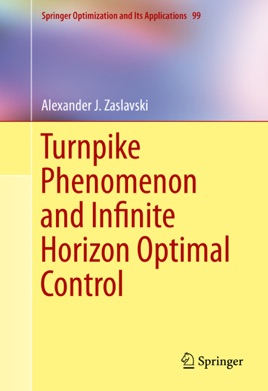• \$84.99

## Publisher Description

This book is devoted to the study of the turnpike phenomenon and describes the existence of solutions for a large variety of infinite horizon optimal control classes of problems.  Chapter 1 provides introductory material on turnpike properties. Chapter 2 studies the turnpike phenomenon for discrete-time optimal control problems. The turnpike properties of autonomous problems with extended-value intergrands are studied in Chapter 3. Chapter 4 focuses on large classes of infinite horizon optimal control problems without convexity (concavity) assumptions. In Chapter 5, the turnpike results for a class of dynamic discrete-time two-player zero-sum game are proven.

This thorough exposition will be very useful  for mathematicians working in the fields of optimal control, the calculus of variations, applied functional analysis, and infinite horizon optimization. It may also be used as a primary text in a graduate course in optimal control or as supplementary text for a variety of courses in other disciplines. Researchers in other fields such as economics and game theory, where turnpike properties are well known, will also find this Work valuable.